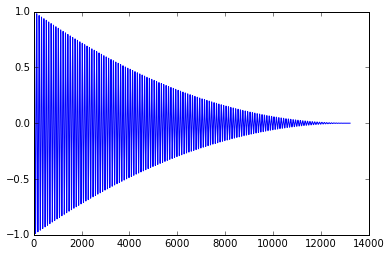# Sounds as Vectors

In :
import numpy as np
import matplotlib.pyplot as pt

from html5_audio import DEFAULT_RATE, get_html5_wave_player


Here's a function that produces a 'beep' with a parabola as an 'envelope':

In :
def make_beep(freq, duration=0.3):
nsamples = DEFAULT_RATE * duration

t = np.linspace(0, duration, nsamples)
data = np.sin(freq*2*np.pi*t)

ramp = np.linspace(0, 1, nsamples)
data = data * (1-ramp)**2

return data


Let's put one into the variable beep:

In :
beep = make_beep(440)


It's just a numpy array:

In :
type(beep)

Out:
numpy.ndarray


A pretty long one though:

In :
beep.shape

Out:
(13230,)


Let's take a look:

In :
pt.plot(beep)

Out:
[<matplotlib.lines.Line2D at 0x7f460b6ba160>]This consists of 44100 'samples' (=data points) per second of sound.

In :
get_html5_wave_player(beep)

Out:

Can we compose some simple 'music' with this? Let's shoot for three seconds...

In :
music = np.zeros(DEFAULT_RATE * 3, dtype=np.float32)

In :
beep1 = make_beep(880, duration=0.8)
beep2 = make_beep(880 * 1.5, duration=0.8)
beep3 = make_beep(880 * 2, duration=0.8)
l = len(beep1)

In :
music.fill(0)
music[0:l] += beep1
s = int(0.3*DEFAULT_RATE)
music[s:s+l] += 0.1*beep2
s = int(0.6*DEFAULT_RATE)
music[s:s+l] += 0.5*beep3

In :
get_html5_wave_player(0.3*music)

Out:
In []: